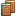##FREQUENTLY ASKED QUESTIONS

How is my rating calculated for multiplayer games?
The ratings system is based on statistical analysis using the Elo formula with a fixed K=10. Here is the formula we use:
 R'A = RA + 10(S-E) where RA is your current rating, S is the score (0=loss or 1=win) and E = 1 1 + 10(RMB - RMA)/400 RMA = RA - 100(M-1)S RMB = RB - 100(M-1)(1-S) where M is the multiplier (doubling cube value multiplied by 1=regular game, 2=gammon, or 3=backgammon), and RB is your opponent's current rating

Essentially, winning against a lower rated opponent yields a small rating increase but losing against a lower rated opponent yields a large rating decrease. Conversely, winning against a higher rated opponent yields a large rating increase but losing against a higher rated opponent yields a small rating decrease. In the case of the doubling cube, winning against a player with the same rating and the final cube value at 4 yields the same rating increase as winning a non-cube game against a player with a rating 400 points above yours.